# Math Behind Software

Scala Software Engineer à SoftwareMill
30 May 2023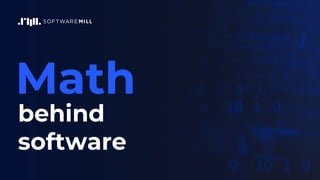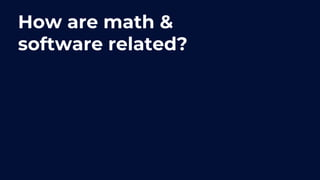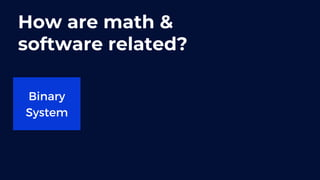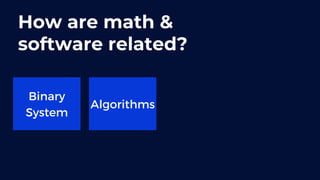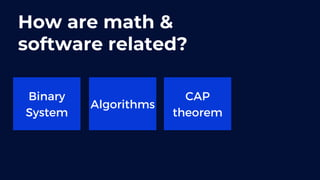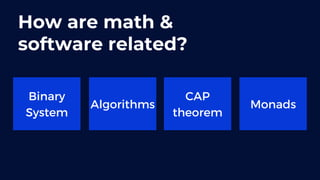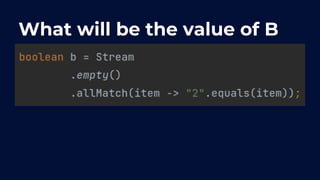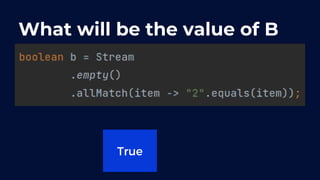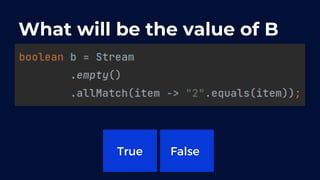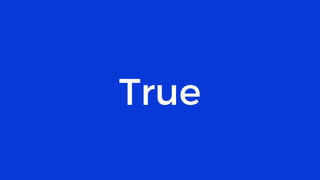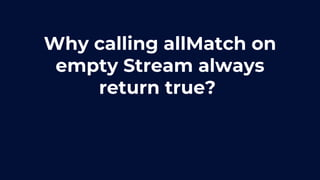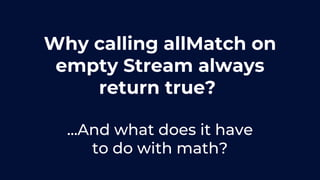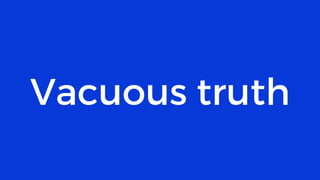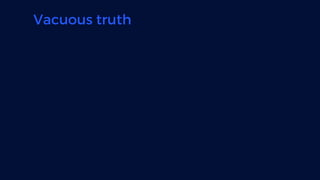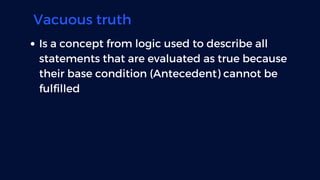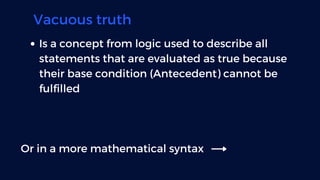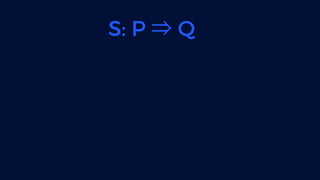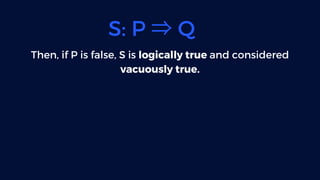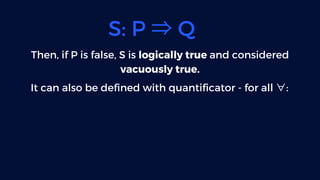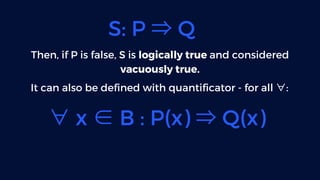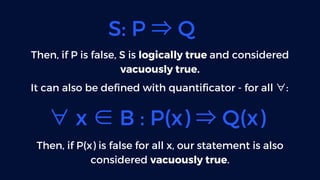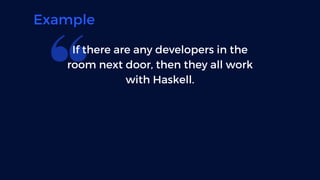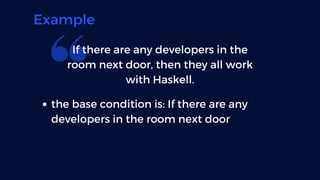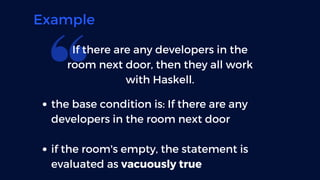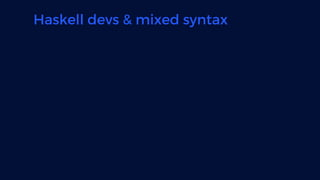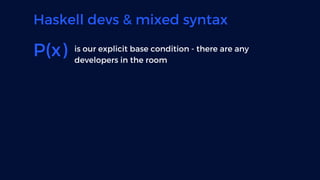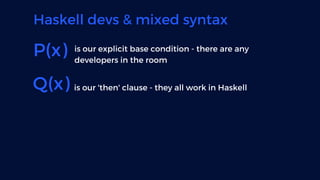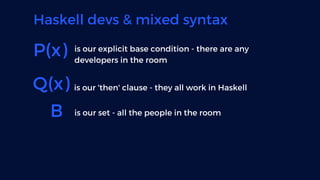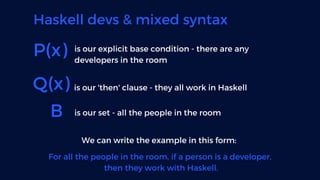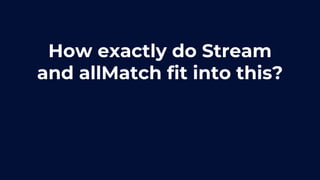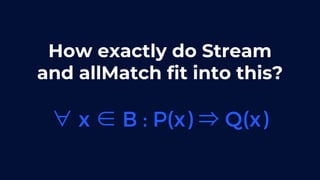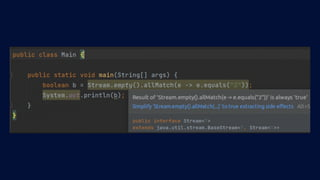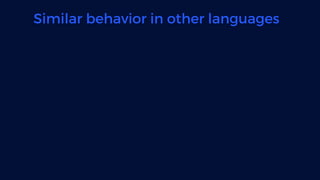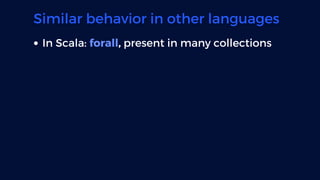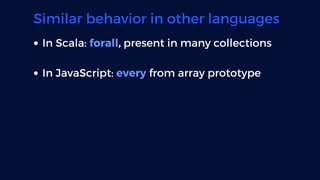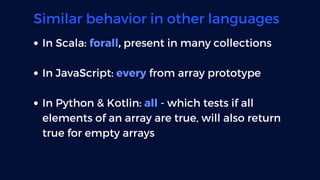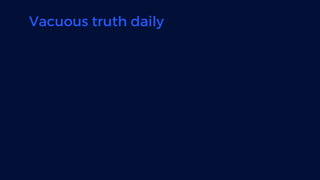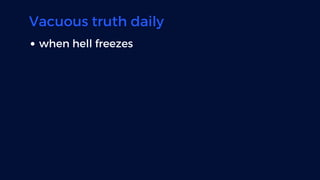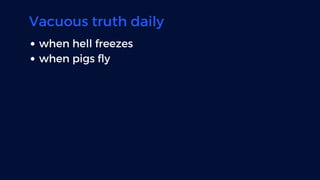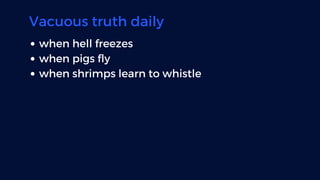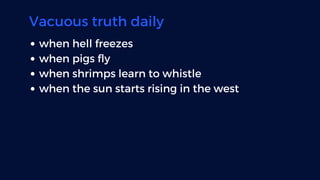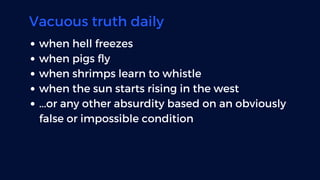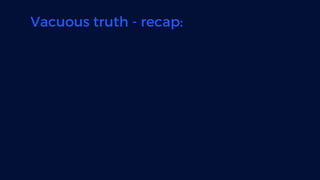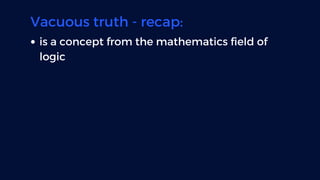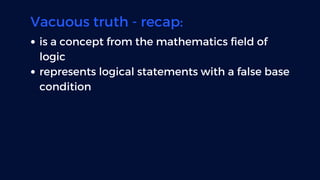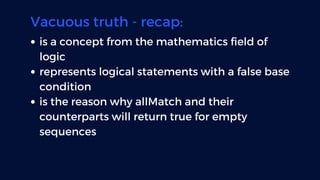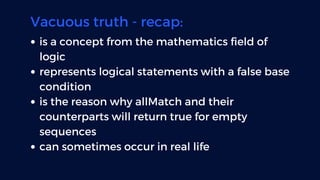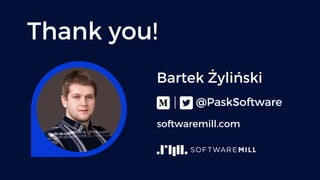1 sur 48

### Math Behind Software

• 2. How are math & software related?
• 3. How are math & software related? Binary System
• 4. How are math & software related? Binary System Algorithms
• 5. How are math & software related? Binary System Algorithms CAP theorem
• 6. How are math & software related? Binary System Algorithms CAP theorem Monads
• 7. What will be the value of B
• 8. What will be the value of B True
• 9. What will be the value of B True False
• 10. True
• 11. Why calling allMatch on empty Stream always return true?
• 12. Why calling allMatch on empty Stream always return true? ...And what does it have to do with math?
• 15. Vacuous truth Is a concept from logic used to describe all statements that are evaluated as true because their base condition (Antecedent) cannot be fulfilled
• 16. Vacuous truth Is a concept from logic used to describe all statements that are evaluated as true because their base condition (Antecedent) cannot be fulfilled Or in a more mathematical syntax
• 18. S: P ⇒Q Then, if P is false, S is logically true and considered vacuously true.
• 19. S: P ⇒Q Then, if P is false, S is logically true and considered vacuously true. It can also be defined with quantificator - for all ∀:
• 20. S: P ⇒Q Then, if P is false, S is logically true and considered vacuously true. It can also be defined with quantificator - for all ∀: ∀x ∈B : P(x) ⇒Q(x)
• 21. S: P ⇒Q Then, if P is false, S is logically true and considered vacuously true. It can also be defined with quantificator - for all ∀: ∀x ∈B : P(x) ⇒Q(x) Then, if P(x) is false for all x, our statement is also considered vacuously true.
• 22. Example If there are any developers in the room next door, then they all work with Haskell.
• 23. Example If there are any developers in the room next door, then they all work with Haskell. the base condition is: If there are any developers in the room next door
• 24. Example If there are any developers in the room next door, then they all work with Haskell. the base condition is: If there are any developers in the room next door if the room's empty, the statement is evaluated as vacuously true
• 25. Haskell devs & mixed syntax
• 26. Haskell devs & mixed syntax P(x) is our explicit base condition - there are any developers in the room
• 27. Haskell devs & mixed syntax P(x) is our explicit base condition - there are any developers in the room Q(x) is our 'then' clause - they all work in Haskell
• 28. Haskell devs & mixed syntax P(x) is our explicit base condition - there are any developers in the room Q(x) is our 'then' clause - they all work in Haskell B is our set - all the people in the room
• 29. Haskell devs & mixed syntax P(x) is our explicit base condition - there are any developers in the room Q(x) is our 'then' clause - they all work in Haskell B is our set - all the people in the room We can write the example in this form: For all the people in the room, if a person is a developer, then they work with Haskell.
• 30. How exactly do Stream and allMatch fit into this?
• 31. How exactly do Stream and allMatch fit into this? ∀x ∈B : P(x) ⇒Q(x)
• 33. Similar behavior in other languages
• 34. Similar behavior in other languages In Scala: forall, present in many collections
• 35. Similar behavior in other languages In Scala: forall, present in many collections In JavaScript: every from array prototype
• 36. Similar behavior in other languages In Scala: forall, present in many collections In JavaScript: every from array prototype In Python & Kotlin: all - which tests if all elements of an array are true, will also return true for empty arrays
• 38. Vacuous truth daily when hell freezes
• 39. Vacuous truth daily when hell freezes when pigs fly
• 40. Vacuous truth daily when hell freezes when pigs fly when shrimps learn to whistle
• 41. Vacuous truth daily when hell freezes when pigs fly when shrimps learn to whistle when the sun starts rising in the west
• 42. Vacuous truth daily when hell freezes when pigs fly when shrimps learn to whistle when the sun starts rising in the west ...or any other absurdity based on an obviously false or impossible condition
• 43. Vacuous truth - recap:
• 44. Vacuous truth - recap: is a concept from the mathematics field of logic
• 45. Vacuous truth - recap: is a concept from the mathematics field of logic represents logical statements with a false base condition
• 46. Vacuous truth - recap: is a concept from the mathematics field of logic represents logical statements with a false base condition is the reason why allMatch and their counterparts will return true for empty sequences
• 47. Vacuous truth - recap: is a concept from the mathematics field of logic represents logical statements with a false base condition is the reason why allMatch and their counterparts will return true for empty sequences can sometimes occur in real life